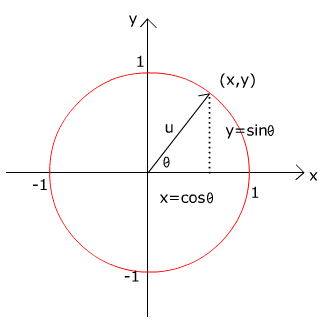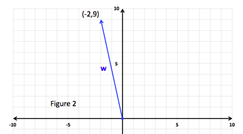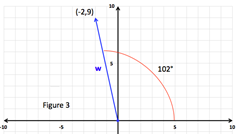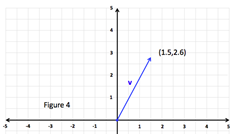# Direction Angles of VectorsFigure 1 shows a unit vector u that makes an angle θ with the positive x-axis. The angle θ is called the directional angle of vector u.

The terminal point of vector u lies on a unit circle and thus u can be denoted by:

Any vector that makes an angle θ with the positive x-axis can be written as the unit vector times the magnitude of the vector.

$v=ai+bj$

Therefore the direction angle of θ of any vector can be calculated as follows:

DIRECTIONAL ANGLE:

Let's look at some examples.

To work these examples requires the use of various vector rules. If you are not familiar with a rule go to the associated topic for a review.

Example 1:Find the direction angle of w = -2i + 9j.
 Step 1: Identify the values for a and b and calculate θ. a = -2, b = 9 $\mathrm{tan}\theta =\frac{b}{a}=\frac{9}{-2}$ $\theta ={\mathrm{tan}}^{-1}|\frac{9}{-2}|$ $\theta \approx 78°$ Step 2: Determine the Quadrant the vector lies in. Because the vector terminus is (-2, 9), it will fall in quadrant II and so will θ.Step 3: Make any necessary adjustments to find the directional angle θ from the positive x-axis. Since the reference angle is 78°, the directional angle from the positive x-axis is 180° - 78° = 102°.Example 2:Find the direction angle of $v=3\left(\mathrm{cos}60°i+\mathrm{sin}60°j\right)$.
 Step 1: Simplify vector v using scalar multiplication. $v=3\left(\mathrm{cos}60°i+\mathrm{sin}60°j\right)$ $v=\frac{3}{2}i+\frac{3\sqrt{3}}{2}$ Step 2: Identify the values for a and b and calculate θ. $\theta ={\mathrm{tan}}^{-1}|\sqrt{3}|$ $\theta =60°$ Step 3: Determine the Quadrant of the vector lies in. Because the vector terminus is and both components are positive the vector will fall in quadrant I and so will θ.Step 4: Make any necessary adjustments to find the directional angle θ from the positive x-axis. Since the reference angle is 60°, the directional angle from the positive x-axis is 60° - 0° = 60°.Related Links: Math algebra The Dot Product Angle between Two Vectors Pre Calculus

To link to this Direction Angles of Vectors page, copy the following code to your site: